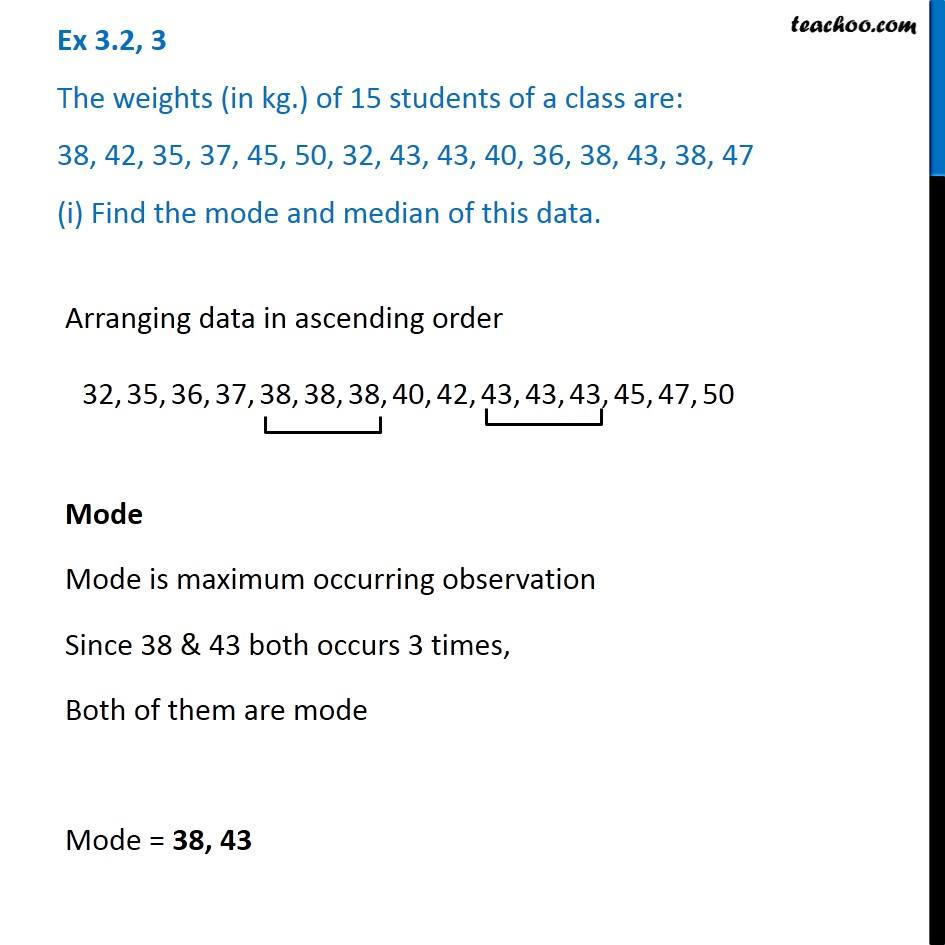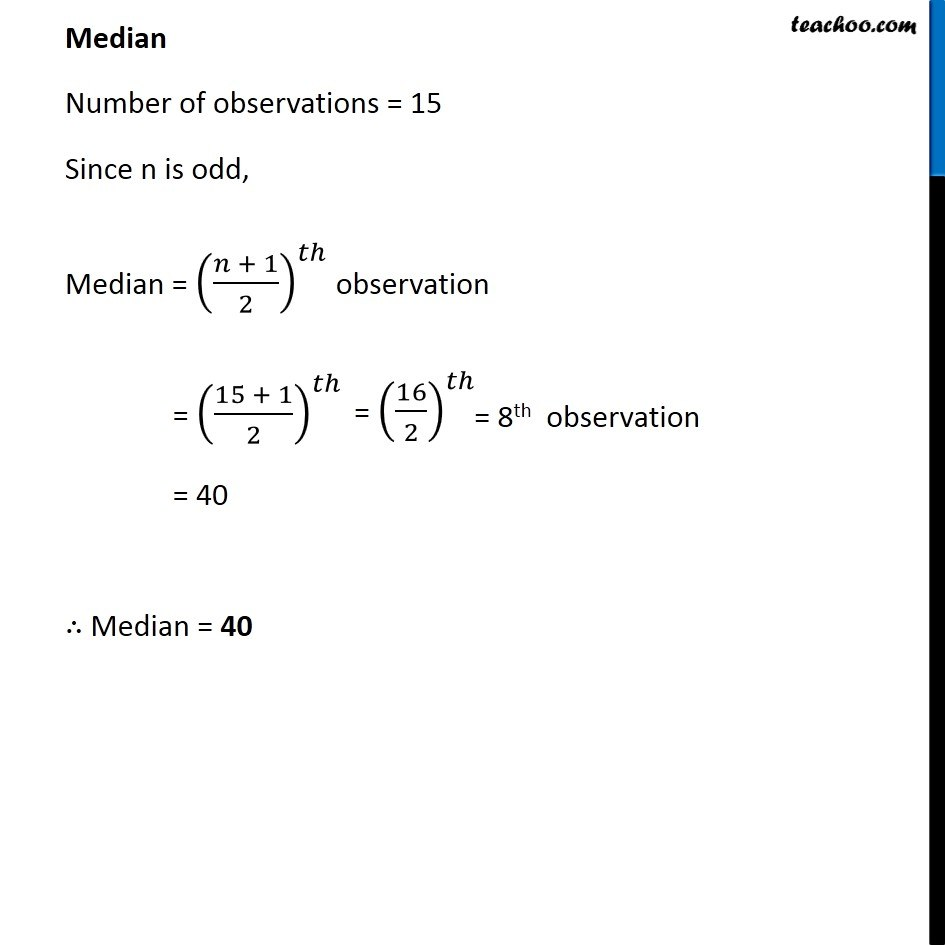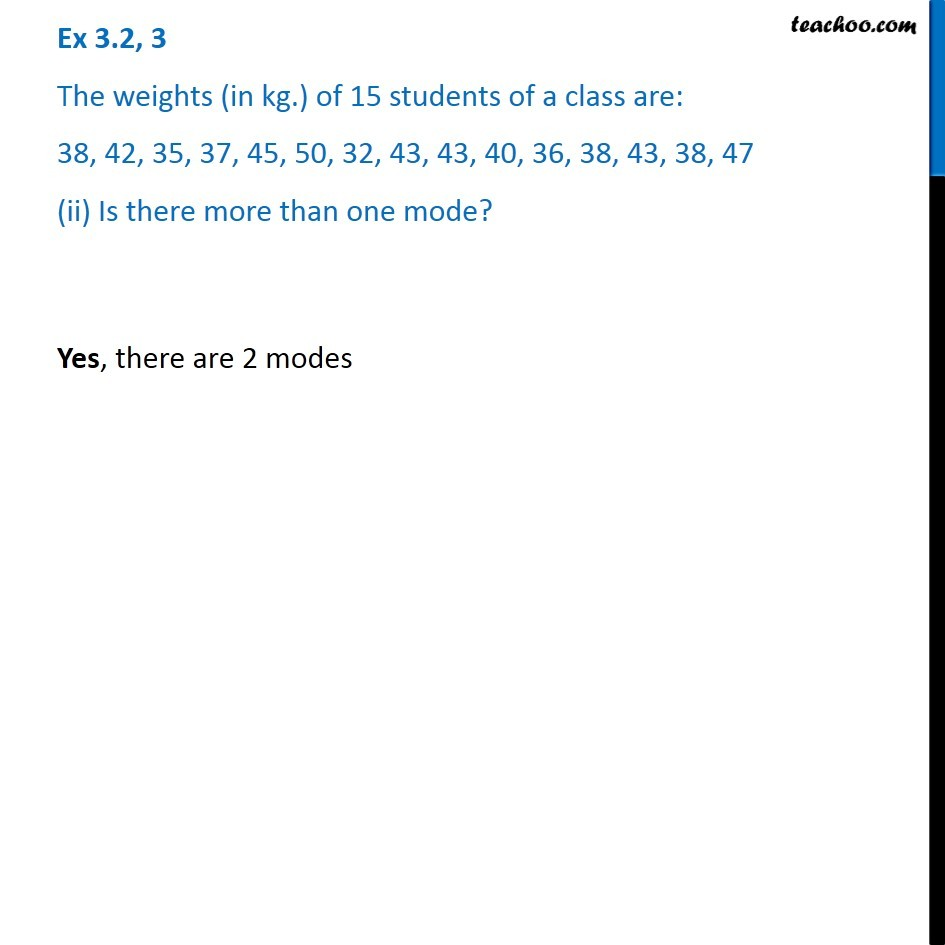Ex 3.2

Chapter 3 Class 7 Data Handling
Serial order wiseLearn in your speed, with individual attention - Teachoo Maths 1-on-1 Class

### Transcript

Ex 3.2, 3 The weights (in kg.) of 15 students of a class are: 38, 42, 35, 37, 45, 50, 32, 43, 43, 40, 36, 38, 43, 38, 47 (i) Find the mode and median of this data. Arranging data in ascending order Mode Mode is maximum occurring observation Since 38 & 43 both occurs 3 times, Both of them are mode Mode = 38, 43 Median Number of observations = 15 Since n is odd, Median = ((𝑛 + 1)/2)^𝑡ℎ observation = ((15 + 1)/2)^𝑡ℎ = 40 ∴ Median = 40 Ex 3.2, 3 The weights (in kg.) of 15 students of a class are: 38, 42, 35, 37, 45, 50, 32, 43, 43, 40, 36, 38, 43, 38, 47 (ii) Is there more than one mode? Yes, there are 2 modes